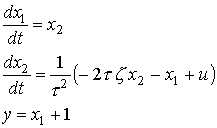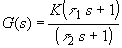CM416
Project No. 4
Due: February 14, 2000 (5pm)
I. Frequency Response Experiment.
Use the simulation located at the URL: http://www.chem.mtu.edu/~tbco/cm416/freq.html .
( Just for your information, the process inside the simulation is given by(1)
with t=3.33 and z=0.2 )
1. Introduce different sinusoidal input into the process and obtain the frequency response data and calculations as you fill the following table:
2.  Input Amplitude Input Frequency Output Amplitude Time Shift Phase Shift Amplitude Ratio Log Modulus Ai wi Bi tshift fi ARi LMi : : : : : : : : : : : : : :

I suggest at least using the following frequency values: 0.1, 0.2, 0.25, 0.27,0.28,0.29,0.3,0.32,0.4,0.5. Also use at least a value of 0.5 for amplitude A.

3. From the table obtained, generate the Bode plots and the Nyquist plots.

4.

II. Bode and Nyquist Plots for Transfer Functions

Using an Excel spreadsheet (or other programs, e.g. MathCad, MATLAB, etc.), generate the Bode Plots and Nyquist Plots for the transfer function:for the following cases:

 Case K t1 t2 1 1 0 0.1 2 1 0 10 3 1 0.1 10 4 1 10 0.1 5 0.01 10 0.1

For a tutorial on the use of Excel for generating the plots, you can link to http://www.chem.mtu.edu/~tbco/cm416/freqexcel.html . For the use of MathCAD, a sample printout of a worksheet can be downloaded in PDF format or Postscript format.

From the Bode plots (both LM vs frequency and Phase Shift vs frequency) obtained, supply the following observations:

1. From case 1 to case 2, how does the corner frequency move with changes in t2 ?
2. From case 2 to case 3, how does a nonzero value of t1 affect the plots ?
3. From case 3 to case 4, how does switching the relative magnitudes of t1 and t2 change the plot ?
4. From case 4 to case 5, how does a decrease in the value of K affect the plots ?

5.
From the Nyquist Plots obtained, supply the following observations:

1. From case 2 to case 3, how does a nonzero value of t1 affect the plots ?
2. From case 3 to case 4, how does switching the relative magnitudes of t1 and t2 change the plot ?
3. From case 4 to case 5, how does a decrease in the value of K affect the plots ?
1. Complex Mapping Theorem
Using the complex mapping program from the link: http://www.chem.mtu.edu/~tbco/cm416/compmap.html , determine the number of clockwise encirclements of the origin that the map of the transfer function  G(s)  make as  s traverse the contour in the clockwise manner, for the following cases:
 Case Contour Zeros Poles 1 Rectangle,  Ax=-0.5,Ay=0.5,  Bx=0.5,By=-0.5 0, -0.2+0.2i, -0.2-0.2i 0.2+0.3i, 0.2-0.3i,-0.8,-1.2 2 same as case 1,  except Ax= -1.5 same as case 1 same as case 1 3 Semicircle,  Ax=0.1, Ay=0,  Bx=0.1, By=0.6 same as case 1 same as case 1 4 Semicircle,  Ax= -0.8, Ay= -0.05,  Bx= -1.0, By=-0.05 same as case 1 same as case 1
(Note: the plots are not able to print out using the print command, so please submit just a rough sketch of the complex maps of each case to show how the mapping is encircling or not encircling the origin. )